# ISEE Lower Level Quantitative : Geometric Translations

## Example Questions

← Previous 1 3 4

### Example Question #1 : Angles And Their Mesures: Ccss.Math.Content.8.G.A.1b

Observe the location of the black and orange angles on the provided coordinate plane and identify which of the following transformations—rotation, translation, or reflection—the black angle has undergone in order to reach the position of the orange angle. Select the answer that provides the correct transformation shown in the provided image.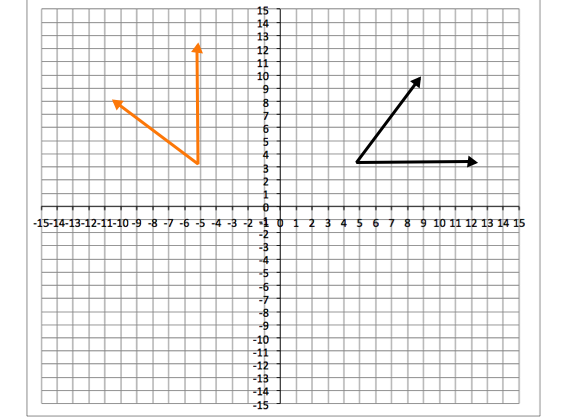A reflection over the x-axis

A translation to the leftrotationrotation

Explanation:

First, let's define the possible transformations.

Rotation: A rotation means turning an image, shape, line, etc. around a central point.

Translation: A translation means moving or sliding an image, shape, line, etc. over a plane.

Reflection: A reflection mean flipping an image, shape, line, etc. over a central line.

In the images from the question, notice that the black angle rotatescounterclockwise, or left around the y-axis. The vertical, base, line of the angle goes from being vertical to horizontal; thus the transformation is a rotation.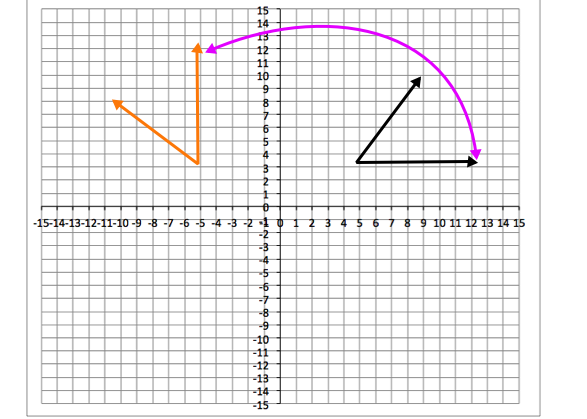The transformation can't be a reflection over the x-axis because the orange angle didn't flip over the x-axis.

The transformation can't be a translation because the angle changes direction, which does not happened when you simply move or slide an angle or image.

### Example Question #1 : Angles And Their Mesures: Ccss.Math.Content.8.G.A.1b

Observe the location of the black and orange angles on the provided coordinate plane and identify which of the following transformations—rotation, translation, or reflection—the black angle has undergone in order to reach the position of the orange angle. Select the answer that provides the correct transformation shown in the provided image.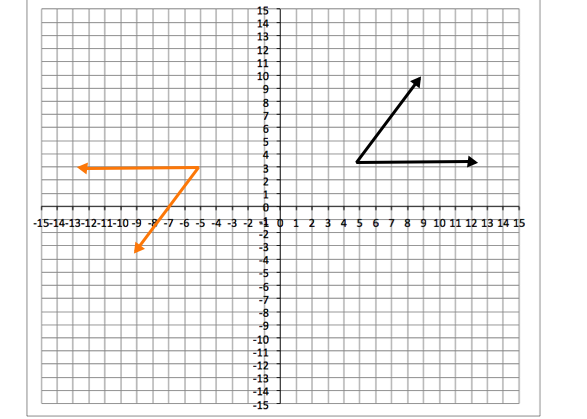rotation

A reflection over the x-axis

A translation to the leftrotation

Explanation:

First, let's define the possible transformations.

Rotation: A rotation means turning an image, shape, line, etc. around a central point.

Translation: A translation means moving or sliding an image, shape, line, etc. over a plane.

Reflection: A reflection mean flipping an image, shape, line, etc. over a central line.

In the images from the question, notice that the black angle rotates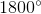counterclockwise, or left around the y-axis. The vertical, base, line of the angle goes from being the base, to the top; thus the transformation is a rotation.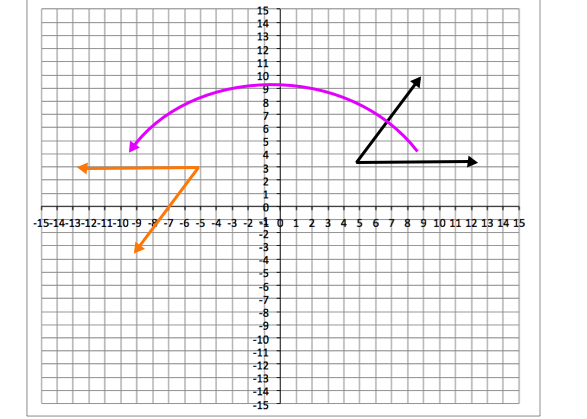The transformation can't be a reflection over the x-axis because the orange angle didn't flip over the x-axis.

The transformation can't be a translation because the angle changes direction, which does not happened when you simply move or slide an angle or image.

### Example Question #3 : Geometric Translations

Observe the location of the black and orange angles on the provided coordinate plane and identify which of the following transformations—rotation, translation, or reflection—the black angle has undergone in order to reach the position of the orange angle. Select the answer that provides the correct transformation shown in the provided image.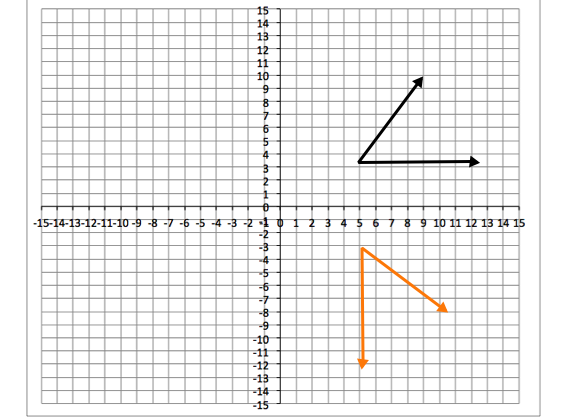A translation down

A reflection over the y-axisrotationrotation

Explanation:

First, let's define the possible transformations.

Rotation: A rotation means turning an image, shape, line, etc. around a central point.

Translation: A translation means moving or sliding an image, shape, line, etc. over a plane.

Reflection: A reflection mean flipping an image, shape, line, etc. over a central line.

In the images from the question, notice that the black angle rotatesclockwise, or right around the x-axis. The vertical, base, line of the angle goes from being vertical to horizontal; thus the transformation is a rotation.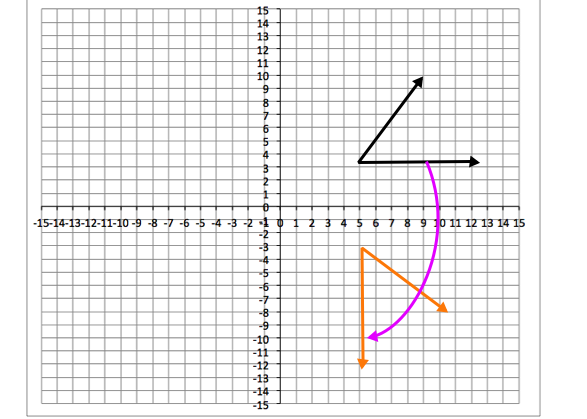The transformation can't be a reflection over the y-axis because the orange angle didn't flip over the y-axis.

The transformation can't be a translation because the angle changes direction, which does not happened when you simply move or slide an angle or image.

### Example Question #4 : Geometric Translations

Observe the location of the black and orange angles on the provided coordinate plane and identify which of the following transformations—rotation, translation, or reflection—the black angle has undergone in order to reach the position of the orange angle. Select the answer that provides the correct transformation shown in the provided image.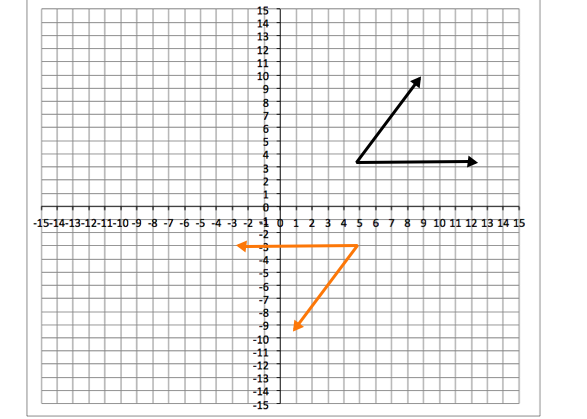A translation down

A reflcetion over the y-axisrotationrotation

Explanation:

First, let's define the possible transformations.

Rotation: A rotation means turning an image, shape, line, etc. around a central point.

Translation: A translation means moving or sliding an image, shape, line, etc. over a plane.

Reflection: A reflection mean flipping an image, shape, line, etc. over a central line.

In the images from the question, notice that the black angle rotatesclockwise, or right around the x-axis. The vertical, base, line of the angle goes from being the base, to the top; thus the transformation is a rotation.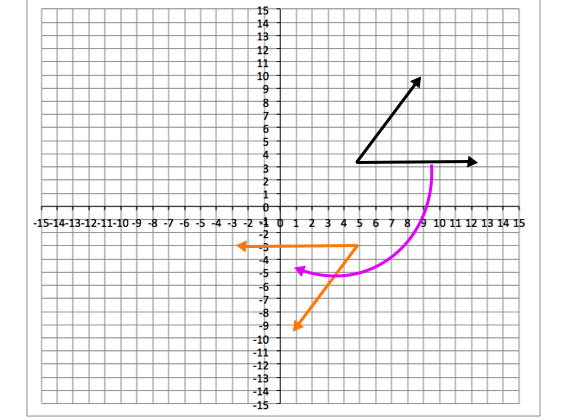The transformation can't be a reflection over the y-axis because the orange angle didn't flip over the y-axis.

The transformation can't be a translation because the angle changes direction, which does not happened when you simply move or slide an angle or image.

### Example Question #331 : Grade 8

Observe the location of the black and orange angles on the provided coordinate plane and identify which of the following transformations—rotation, translation, or reflection—the black angle has undergone in order to reach the position of the orange angle. Select the answer that provides the correct transformation shown in the provided image.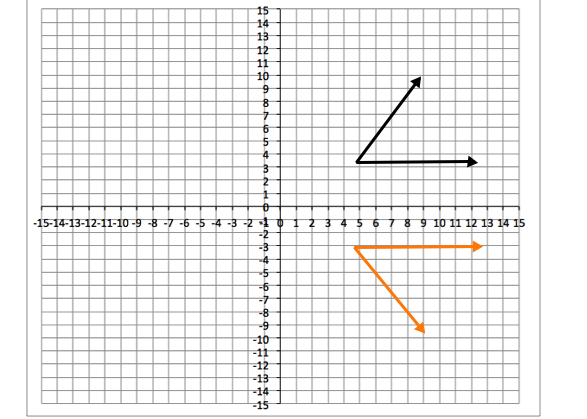Reflection over the x-axis

A translation downrotation

Reflection over the x-axis

Explanation:

First, let's define the possible transformations.

Rotation: A rotation means turning an image, shape, line, etc. around a central point.

Translation: A translation means moving or sliding an image, shape, line, etc. over a plane.

Reflection: A reflection mean flipping an image, shape, line, etc. over a central line.

In the images from the question, the line was not rotatedbecause that rotation would have caused the vertical, base, line of the angle to go from being horizontal to vertical, but the line is still horizontal. The line was not moved down, as the translation is described in the answer choice, because you can tell the angle has been flipped, the straight, base line of the angle is now the top line of the angle; thus, the correct answer is a reflection over the x-axis.

### Example Question #6 : Geometric Translations

Observe the location of the black and orange angles on the provided coordinate plane and identify which of the following transformations—rotation, translation, or reflection—the black angle has undergone in order to reach the position of the orange angle. Select the answer that provides the correct transformation shown in the provided image.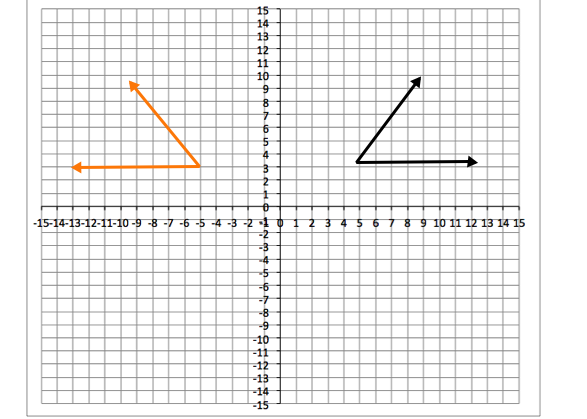rotation

A translation left

Reflection over the y-axis

Reflection over the y-axis

Explanation:

First, let's define the possible transformations.

Rotation: A rotation means turning an image, shape, line, etc. around a central point.

Translation: A translation means moving or sliding an image, shape, line, etc. over a plane.

Reflection: A reflection mean flipping an image, shape, line, etc. over a central line.

In the images from the question, the line was not rotatedbecause that rotation would have caused the vertical, base, line of the angle to go from being horizontal to vertical, but the line is still horizontal. The line was not moved to the left, as the translation is described in the answer choice, because you can tell the angle has been flipped, the opening of the angle is facing the opposite direction; thus, the correct answer is a reflection over the y-axis.

### Example Question #7 : Geometric Translations

Observe the location of the black and orange angles on the provided coordinate plane and identify which of the following transformations—rotation, translation, or reflection—the black angle has undergone in order to reach the position of the orange angle. Select the answer that provides the correct transformation shown in the provided image.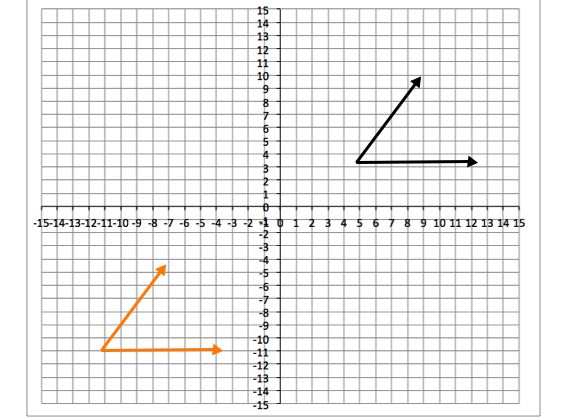rotation

A reflection over the y-axis

A translation down and at a diagonal

A translation down and at a diagonal

Explanation:

First, let's define the possible transformations.

Rotation: A rotation means turning an image, shape, line, etc. around a central point.

Translation: A translation means moving or sliding an image, shape, line, etc. over a plane.

Reflection: A reflection mean flipping an image, shape, line, etc. over a central line.

In the images from the question, the line was not rotatedbecause that rotation would have caused the vertical, base, line of the angle to go from being horizontal to vertical, but the line is still horizontal. The line was not reflected over the y-axis because the angle was not flipped and the opening of the angle is not facing the opposite direction; thus, the correct answer is a translation down and at diagonal.

### Example Question #8 : Geometric Translations

Observe the location of the black and orange angles on the provided coordinate plane and identify which of the following transformations—rotation, translation, or reflection—the black angle has undergone in order to reach the position of the orange angle. Select the answer that provides the correct transformation shown in the provided image.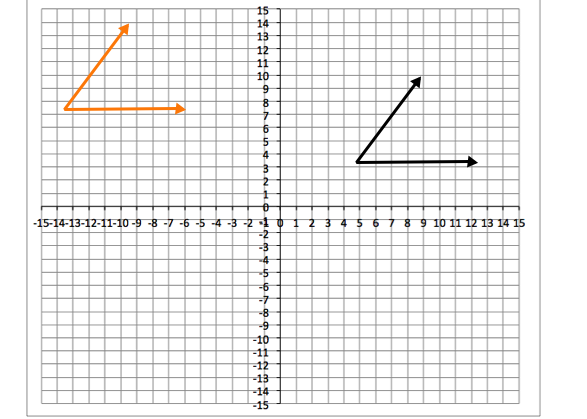rotation

A reflection over the y-axis

A translation up and to the left

A translation up and to the left

Explanation:

First, let's define the possible transformations.

Rotation: A rotation means turning an image, shape, line, etc. around a central point.

Translation: A translation means moving or sliding an image, shape, line, etc. over a plane.

Reflection: A reflection mean flipping an image, shape, line, etc. over a central line.

In the images from the question, the line was not rotatedbecause that rotation would have caused the vertical, base, line of the angle to go from being horizontal to vertical, but the line is still horizontal. The line was not reflected over the y-axis because the angle was not flipped and the opening of the angle is not facing the opposite direction; thus, the correct answer is a translation up and to the left.

### Example Question #9 : Geometric Translations

Observe the location of the black and orange angles on the provided coordinate plane and identify which of the following transformations—rotation, translation, or reflection—the black angle has undergone in order to reach the position of the orange angle. Select the answer that provides the correct transformation shown in the provided image.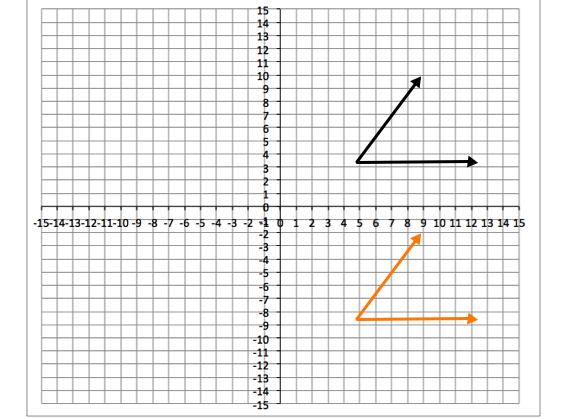rotation

Translation down

A reflection over the x-axis

Translation down

Explanation:

First, let's define the possible transformations.

Rotation: A rotation means turning an image, shape, line, etc. around a central point.

Translation: A translation means moving or sliding an image, shape, line, etc. over a plane.

Reflection: A reflection mean flipping an image, shape, line, etc. over a central line.

In the images from the question, the line was not rotatedbecause that rotation would have caused the vertical, base, line of the angle to go from being horizontal to vertical, but the line is still horizontal. The line was not reflected over the x-axis because the angle was not flipped and the base of the angle is the straight line, like that of the black angle; thus, the correct answer is a translation down.

### Example Question #10 : Geometric Translations

Observe the location of the black and orange angles on the provided coordinate plane and identify which of the following transformations—rotation, translation, or reflection—the black angle has undergone in order to reach the position of the orange angle. Select the answer that provides the correct transformation shown in the provided image.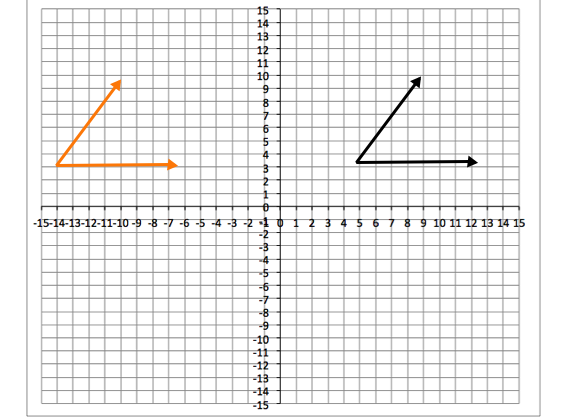A reflection over the y-axis

Translation to the left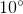rotation

Translation to the left

Explanation:

First, let's define the possible transformations.

Rotation: A rotation means turning an image, shape, line, etc. around a central point.

Translation: A translation means moving or sliding an image, shape, line, etc. over a plane.

Reflection: A reflection mean flipping an image, shape, line, etc. over a central line.

In the images from the question, the line was not rotatedbecause that rotation would have caused direction of the angle to turn a bit, but the angle's direction did not change. The line was not reflected over the y-axis because the angle was not flipped and the opening of the angle is not facing the opposite direction; thus, the correct answer is a translation to the left.

← Previous 1 3 4